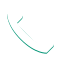# Depreciation Recapture Example | How to Avoid With the 1031 Exchange

Last updated on June 23rd, 2021 at 02:59 pm

A Sample Calculation of Depreciation Recapture Tax

The primary reason why prudent real estate investors use a 1301 exchange is to lower their tax liability in a given year. Essentially, this tax-sheltering mechanism involves an investor exchanging one property for another like-kind property without incurring any tax liability from the exchange, unlike many other investment swaps that are subject to taxes. We’ll give a depreciation recapture example that will help illustrate just how helpful 1031 exchanges are.

Through this exchange, many investors are able to defer their depreciation recapture tax liability until their passing, which triggers a “stepped up” basis for their heir’s inherited property.

Before walking through a depreciation recapture example from the sale of a rental property, a general understanding of some of the core concepts related to the 1031 exchange and depreciation recapture will be helpful.

## What is Depreciation Recapture Tax?

### Understanding Depreciation

Depreciation is the method of calculating a tax deduction for the costs involved in purchasing and improving upon the building of a rental property. Given that depreciation does not necessarily reflect specific cash expenditures in a tax year, the tax deduction results in a cash boost to the investor.

### How Depreciation Recapture Works

Without a specific tax write-off for an actual expense incurred in maintaining or improving upon the property, the depreciation tax deduction is far too generous to the investor from the perspective of the IRS. Depreciation recapture is how the IRS prevents real estate investors from seemingly exploiting the depreciation tax deduction for their property.

At the point of sale of a property, the IRS collects a real estate depreciation recapture tax based on the adjusted cost basis of the property. This is calculated by subtracting the amount of the depreciation deduction taken each tax year from the cost basis of the property. We have a helpful depreciation recapture calculator if you are thinking about selling your rental property.

Keep in mind that the values are based on the physical structure’s value without including the cost of the land itself. The tax rate for depreciation recapture is based on the investor’s income tax rate and is owed in addition to any capital gains taxes incurred from the sale of the property.

Now, consider the following depreciation recapture example to get a sense of how important it could be for you to defer the depreciation recapture tax by taking advantage of the 1031 exchange.

## Depreciation Recapture Example for a Sample Rental Property Investment

In this example, we’ll explore how the depreciation recapture is calculated for a residential investment property. Let’s say that your investment property is a single-family townhouse that you purchased as an investment five years ago for \$250,000. The value of the building was \$200,000.

If you divide the original building value by 27.5 years, the IRS’s useful life designation for residential investment properties, then your annual depreciation is \$7,272.72.

Now, you just sold the property for \$300,000. Since you held the property for five years, we multiply that by the \$7,272.72 of annual depreciation to calculate the total depreciation tax expense of \$36,363.63.

This means that your adjusted cost basis is \$213,636.37. You will be taxed on \$86,363.63 of gain. For the depreciation recapture of \$36,363.63, you would owe tax at your specific income tax rate. For example, at an income tax rate of 25%, you would owe \$9,090.90. The remaining \$50,000 of gain would be taxed at the lower capital gains rate of up to 15%.

## Next Steps to Defer the Depreciation Recapture Tax

No real estate investor wants to be stuck with an unexpected tax liability upon selling their property. The deferral mechanism of the 1031 like-kind exchange is a powerful tool when used properly to avoid triggering the depreciation recapture tax. A knowledgeable accountant can assess your investment scenario to help navigate the complexities of the 1031 exchange regulations.Call Now (949) 409-6585‬